All submissions of the EM system will be redirected to Online Manuscript Submission System. Authors are requested to submit articles directly to Online Manuscript Submission System of respective journal.

# The Basis of Quantum Mathematics

Department of Mathematics, The University of Azarabadegan, Urmia, Iran

*Corresponding Author:
Department of Mathematics,
Urmia,
Iran

Received: 09-May-2022, Manuscript No. JSMS-22-65556; Editor assigned: 13-May-2022, Pre QC No. JSMS-22-65556 (PQ); Reviewed: 31-May-2022, QC No. JSMS-22-65556; Revised: 07-Jun-2022, Manuscript No. JSMS-22-65556 (A); Published: 14-Jun-2022, DOI: 10.4172/J Stats Math Sci.8.5.002.

Visit for more related articles at Research & Reviews: Journal of Statistics and Mathematical Sciences

## Abstract

In quantum computer, due to the existing parameters, such as gravity, time, speed of light, etc., a digital computer cannot be used, and to determine the above parameters, you have to go through a tortuous path, heavy cost and a long time, To produce a quantum computer and sell it at a high price. Therefore, if the above parameters are specified in terms of number=c1 (x, y, c1) for preparing a quantum computer, digital computers can also be used for quantum basic computers.

#### Keywords

Quantum mathematics; Gravity triangle; Fifth dimension; Stable dynamics; Light speed triangle

#### Description

Description of defining the parameters

We know that the function of a quantum computer is to study micro-properties such as electrons, neutrons, etc. Specifications of fine particles include:

• Particle speed equal to the speed of light.
• Gravity around the center point.

If we can define the above two factors based on (x, y, c1) in digital computer, quantum computer can operate based on digital computer performance and be available to everyone as soon as possible, cheap cost and desired selling price.

#### Abbreviations/Symbols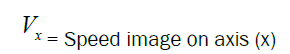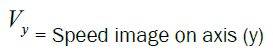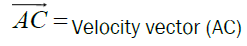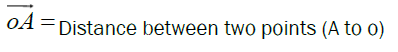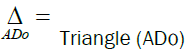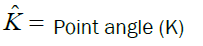Continuation of mathematical operations: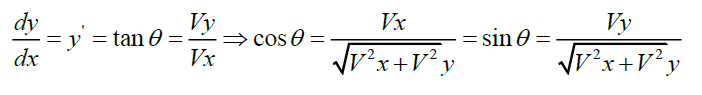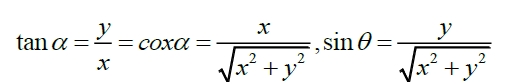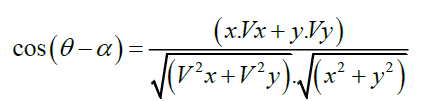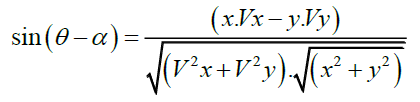.

Before proving the "basics of quantum mathematics" it is necessary to explain the basics of digital computer mathematics and then to distinguish between digital computer mathematics and quantum computer mathematics. As described in the summary, in a digital computer all inputs must be defined as (numbers, y and x) to be meaningful to the computer and all software must be defined as above. In quantum computer, all related problems and obstacles such as gravity, time, speed of light, etc. should be eliminated and presented as (numbers, y and x). Therefore, it is necessary to observe the following:

Gravity

We know that all the tiny particles in the universe, which is part of the universe, are moving. This type of motion is moving according to the laws (physics, mechanics and mathematics). This type of law has been proven by the law of stable dynamics or the fifth dimension.

The image of the fifth dimension in Figure 1 (a) is drawn as a triangle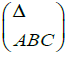and the vector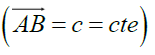The vector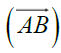is the moving velocity vector of point (A) on the moving tangent to the curve at

Point (A). It is worth noting that in a triangle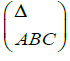the vector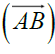is the chord of the triangle and is always equal to the speed of light, and this fixed number is defined for the computer. That’s mean: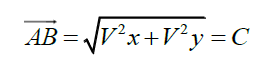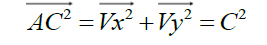Quoted from the fifth dimension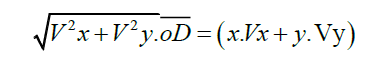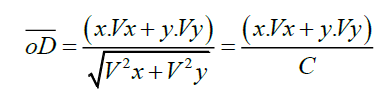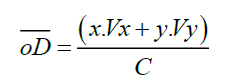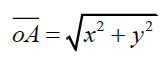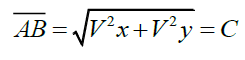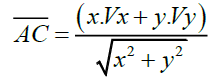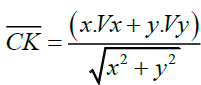We now have two triangles of velocity and distance:

Speed triangle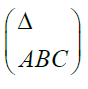Distance triangle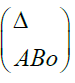If we compare these two triangles, we can define the gravitational problem, gravity and speed of light as numbers (numbers, y and x) for the computer. To compare the two triangles, it is sufficient to draw a line parallel to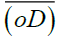from point (c) in triangle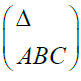to intersect the line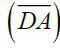at point (K). That the two triangles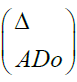and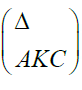are similar and therefore we will have: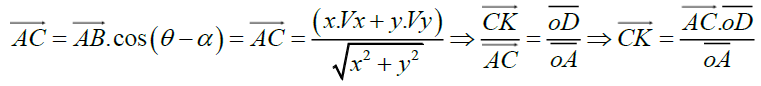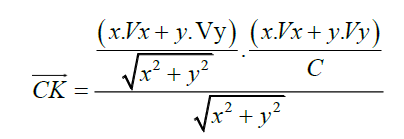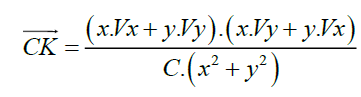Now that the above relationships and the form of the curve problem are specified, the relevant software for the quantum computer can be defined.

Method 1: A dual-core CPU, the first core is tracked through a right triangle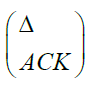and based on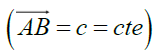and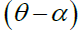angel and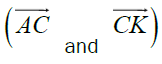and the second core (CPU) based on the triangle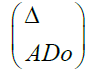(perpendicular). And based on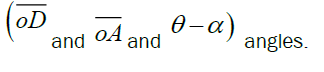The second method: a two-core CPU, the first core through the coordinates of point (D) and two sides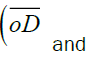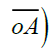and the second core through the coordinates of point (D) and three sides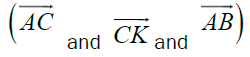and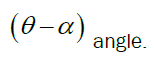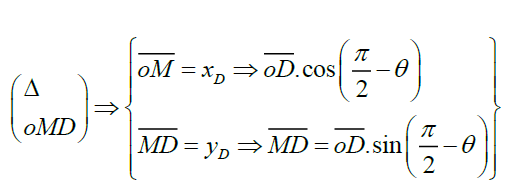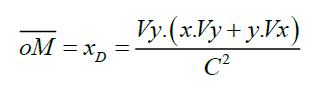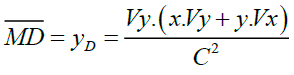Method 3: In this method, it is suggested to use several dual-core CPUs and to use all the rules (physics, mechanics and mathematics available for different applications.

In order to complete quantum software in all the laws of physics, mechanics and mathematics, it is necessary to use several (CPU). One or two or four, or eight or sixteen or thirty-two or sixty-four, etc., in the form of two cores, depending on the volume of operations.